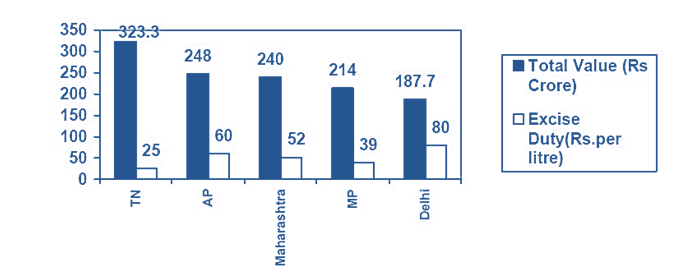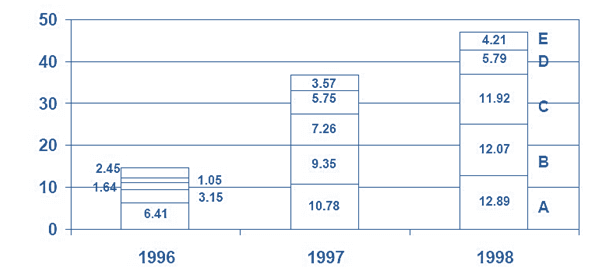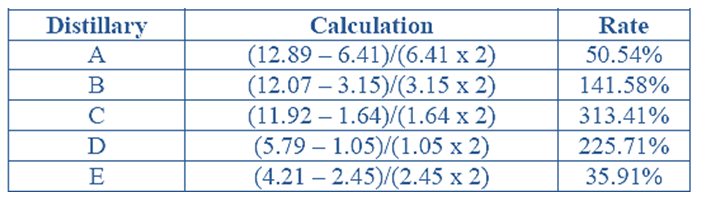Direction for the questions: The following graph shows the value of liquor supplied by the 5 states in 1996 and the excise duty rates in each state.Amount of liquor supplied in Tamil Nadu Distilleries A, B, C, D, E (from bottom to top) in lakh litres.Question 1: What is the lowest percentage difference in the excise duty rates for any two states (take base as the higher duty value)?
(a) 13.33%
(b) 15
(c) 25
(d) Cannot be determined.

Question 2: Which of the five states manufactured liquor at the lowest cost?
(b) Delhi
(c) The states which has the lowest value for (wholesale price-Excise duty) per litre
(d) Cannot be determined.

Question 3: If Excise duty is levied before the goods leave the factory (on the value of the liquor), then which of the following choices shows distilleries in ascending order of the excise duty paid by them for the year 1996? (Assume the total liquor in TN is supplied by only these 5 distilleries).
(a) ECABD
(c) DCEBA
(d) Cannot be determined.

Question 4: If the Tamil Nadu distillery, with the least average simple annual growth in amount of liquor supplied in the given period had shown the same rate of growth as the one which grew fastest, what would that company’s supply have been in 1998, in lakh liters?
(a) 13
(b) 15.11
(c) 130
(d) Cannot be determined.

Answer 1. a We can see that the lowest difference in values is between the rates of AP and Maharashtra. This difference of Rs. 8 per litre, in percentage terms, is 13.33%. Remember, as stated in the question, we take 60 as base here.

Answer 2. d As we are only given the total value and we are not provided the figures for the total amount of liquor manufactured, we cannot calculate the cost. Thus, the correct answer is option (d).

Answer 3. c It is given that the Excise duty is levied on the total value of liquor produced. Now, it is common sense that the duty collected with the same as the order as the order of the amount of the liquor produced by them (as the excise duty rate remains constant). Thus, the correct order is DCEBA.

Answer 4. b The first step is to calculate the growth rates in this case.

These are tabulated below for the five distillers=As we can see that the distillery with highest growth rate is C

Also, we can see that the distillery with lowest growth rate is E.

Now we must take the growth rate of E grown as 313.41% for the2-year period.

Thus, the overall its supply in 1998 would be (2.45 x 616.82 /100) = 15.11 liters.